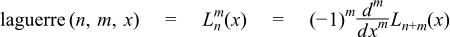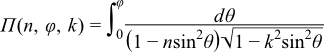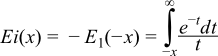#Boost C++ Libraries

...one of the most highly regarded and expertly designed C++ library projects in the world.

This is the documentation for an old version of boost. Click here for the latest Boost documentation.

#### TR1 C Functions Quick Reference

##### Supported TR1 Functions
```namespace boost{ namespace math{ namespace tr1{ extern "C"{

// [5.2.1.1] associated Laguerre polynomials:
double assoc_laguerre(unsigned n, unsigned m, double x);
float assoc_laguerref(unsigned n, unsigned m, float x);
long double assoc_laguerrel(unsigned n, unsigned m, long double x);

// [5.2.1.2] associated Legendre functions:
double assoc_legendre(unsigned l, unsigned m, double x);
float assoc_legendref(unsigned l, unsigned m, float x);
long double assoc_legendrel(unsigned l, unsigned m, long double x);

// [5.2.1.3] beta function:
double beta(double x, double y);
float betaf(float x, float y);
long double betal(long double x, long double y);

// [5.2.1.4] (complete) elliptic integral of the first kind:
double comp_ellint_1(double k);
float comp_ellint_1f(float k);
long double comp_ellint_1l(long double k);

// [5.2.1.5] (complete) elliptic integral of the second kind:
double comp_ellint_2(double k);
float comp_ellint_2f(float k);
long double comp_ellint_2l(long double k);

// [5.2.1.6] (complete) elliptic integral of the third kind:
double comp_ellint_3(double k, double nu);
float comp_ellint_3f(float k, float nu);
long double comp_ellint_3l(long double k, long double nu);

// [5.2.1.8] regular modified cylindrical Bessel functions:
double cyl_bessel_i(double nu, double x);
float cyl_bessel_if(float nu, float x);
long double cyl_bessel_il(long double nu, long double x);

// [5.2.1.9] cylindrical Bessel functions (of the first kind):
double cyl_bessel_j(double nu, double x);
float cyl_bessel_jf(float nu, float x);
long double cyl_bessel_jl(long double nu, long double x);

// [5.2.1.10] irregular modified cylindrical Bessel functions:
double cyl_bessel_k(double nu, double x);
float cyl_bessel_kf(float nu, float x);
long double cyl_bessel_kl(long double nu, long double x);

// [5.2.1.11] cylindrical Neumann functions;
// cylindrical Bessel functions (of the second kind):
double cyl_neumann(double nu, double x);
float cyl_neumannf(float nu, float x);
long double cyl_neumannl(long double nu, long double x);

// [5.2.1.12] (incomplete) elliptic integral of the first kind:
double ellint_1(double k, double phi);
float ellint_1f(float k, float phi);
long double ellint_1l(long double k, long double phi);

// [5.2.1.13] (incomplete) elliptic integral of the second kind:
double ellint_2(double k, double phi);
float ellint_2f(float k, float phi);
long double ellint_2l(long double k, long double phi);

// [5.2.1.14] (incomplete) elliptic integral of the third kind:
double ellint_3(double k, double nu, double phi);
float ellint_3f(float k, float nu, float phi);
long double ellint_3l(long double k, long double nu, long double phi);

// [5.2.1.15] exponential integral:
double expint(double x);
float expintf(float x);
long double expintl(long double x);

// [5.2.1.16] Hermite polynomials:
double hermite(unsigned n, double x);
float hermitef(unsigned n, float x);
long double hermitel(unsigned n, long double x);

// [5.2.1.18] Laguerre polynomials:
double laguerre(unsigned n, double x);
float laguerref(unsigned n, float x);
long double laguerrel(unsigned n, long double x);

// [5.2.1.19] Legendre polynomials:
double legendre(unsigned l, double x);
float legendref(unsigned l, float x);
long double legendrel(unsigned l, long double x);

// [5.2.1.20] Riemann zeta function:
double riemann_zeta(double);
float riemann_zetaf(float);
long double riemann_zetal(long double);

// [5.2.1.21] spherical Bessel functions (of the first kind):
double sph_bessel(unsigned n, double x);
float sph_besself(unsigned n, float x);
long double sph_bessell(unsigned n, long double x);

// [5.2.1.22] spherical associated Legendre functions:
double sph_legendre(unsigned l, unsigned m, double theta);
float sph_legendref(unsigned l, unsigned m, float theta);
long double sph_legendrel(unsigned l, unsigned m, long double theta);

// [5.2.1.23] spherical Neumann functions;
// spherical Bessel functions (of the second kind):
double sph_neumann(unsigned n, double x);
float sph_neumannf(unsigned n, float x);
long double sph_neumannl(unsigned n, long double x);

}}}} // namespaces
```

In addition sufficient additional overloads of the `double` versions of the above functions are provided, so that calling the function with any mixture of `float`, `double`, ```long double```, or integer arguments is supported, with the return type determined by the result type calculation rules.

For example:

```expintf(2.0f);  // float version, returns float.
expint(2.0f);   // also calls the float version and returns float.
expint(2.0);    // double version, returns double.
expintl(2.0L);  // long double version, returns a long double.
expint(2.0L);   // also calls the long double version.
expint(2);      // integer argument is treated as a double, returns double.
```
##### Quick Reference
```// [5.2.1.1] associated Laguerre polynomials:
double assoc_laguerre(unsigned n, unsigned m, double x);
float assoc_laguerref(unsigned n, unsigned m, float x);
long double assoc_laguerrel(unsigned n, unsigned m, long double x);
```

The assoc_laguerre functions return:See also laguerre for the full template (header only) version of this function.

```// [5.2.1.2] associated Legendre functions:
double assoc_legendre(unsigned l, unsigned m, double x);
float assoc_legendref(unsigned l, unsigned m, float x);
long double assoc_legendrel(unsigned l, unsigned m, long double x);
```

The assoc_legendre functions return:See also legendre_p for the full template (header only) version of this function.

```// [5.2.1.3] beta function:
double beta(double x, double y);
float betaf(float x, float y);
long double betal(long double x, long double y);
```

Returns the beta function of x and y:See also beta for the full template (header only) version of this function.

```// [5.2.1.4] (complete) elliptic integral of the first kind:
double comp_ellint_1(double k);
float comp_ellint_1f(float k);
long double comp_ellint_1l(long double k);
```

Returns the complete elliptic integral of the first kind of k:See also ellint_1 for the full template (header only) version of this function.

```// [5.2.1.5] (complete) elliptic integral of the second kind:
double comp_ellint_2(double k);
float comp_ellint_2f(float k);
long double comp_ellint_2l(long double k);
```

Returns the complete elliptic integral of the second kind of k:See also ellint_2 for the full template (header only) version of this function.

```// [5.2.1.6] (complete) elliptic integral of the third kind:
double comp_ellint_3(double k, double nu);
float comp_ellint_3f(float k, float nu);
long double comp_ellint_3l(long double k, long double nu);
```

Returns the complete elliptic integral of the third kind of k and nu:See also ellint_3 for the full template (header only) version of this function.

```// [5.2.1.8] regular modified cylindrical Bessel functions:
double cyl_bessel_i(double nu, double x);
float cyl_bessel_if(float nu, float x);
long double cyl_bessel_il(long double nu, long double x);
```

Returns the modified bessel function of the first kind of nu and x:See also cyl_bessel_i for the full template (header only) version of this function.

```// [5.2.1.9] cylindrical Bessel functions (of the first kind):
double cyl_bessel_j(double nu, double x);
float cyl_bessel_jf(float nu, float x);
long double cyl_bessel_jl(long double nu, long double x);
```

Returns the bessel function of the first kind of nu and x:See also cyl_bessel_j for the full template (header only) version of this function.

```// [5.2.1.10] irregular modified cylindrical Bessel functions:
double cyl_bessel_k(double nu, double x);
float cyl_bessel_kf(float nu, float x);
long double cyl_bessel_kl(long double nu, long double x);
```

Returns the modified bessel function of the second kind of nu and x:See also cyl_bessel_k for the full template (header only) version of this function.

```// [5.2.1.11] cylindrical Neumann functions;
// cylindrical Bessel functions (of the second kind):
double cyl_neumann(double nu, double x);
float cyl_neumannf(float nu, float x);
long double cyl_neumannl(long double nu, long double x);
```

Returns the bessel function of the second kind (Neumann function) of nu and x:See also cyl_neumann for the full template (header only) version of this function.

```// [5.2.1.12] (incomplete) elliptic integral of the first kind:
double ellint_1(double k, double phi);
float ellint_1f(float k, float phi);
long double ellint_1l(long double k, long double phi);
```

Returns the incomplete elliptic integral of the first kind of k and phi:See also ellint_1 for the full template (header only) version of this function.

```// [5.2.1.13] (incomplete) elliptic integral of the second kind:
double ellint_2(double k, double phi);
float ellint_2f(float k, float phi);
long double ellint_2l(long double k, long double phi);
```

Returns the incomplete elliptic integral of the second kind of k and phi:See also ellint_2 for the full template (header only) version of this function.

```// [5.2.1.14] (incomplete) elliptic integral of the third kind:
double ellint_3(double k, double nu, double phi);
float ellint_3f(float k, float nu, float phi);
long double ellint_3l(long double k, long double nu, long double phi);
```

Returns the incomplete elliptic integral of the third kind of k, nu and phi:See also ellint_3 for the full template (header only) version of this function.

```// [5.2.1.15] exponential integral:
double expint(double x);
float expintf(float x);
long double expintl(long double x);
```

Returns the exponential integral Ei of x:See also expint for the full template (header only) version of this function.

```// [5.2.1.16] Hermite polynomials:
double hermite(unsigned n, double x);
float hermitef(unsigned n, float x);
long double hermitel(unsigned n, long double x);
```

Returns the n'th Hermite polynomial of x:See also hermite for the full template (header only) version of this function.

```// [5.2.1.18] Laguerre polynomials:
double laguerre(unsigned n, double x);
float laguerref(unsigned n, float x);
long double laguerrel(unsigned n, long double x);
```

Returns the n'th Laguerre polynomial of x:See also laguerre for the full template (header only) version of this function.

```// [5.2.1.19] Legendre polynomials:
double legendre(unsigned l, double x);
float legendref(unsigned l, float x);
long double legendrel(unsigned l, long double x);
```

Returns the l'th Legendre polynomial of x:See also legendre_p for the full template (header only) version of this function.

```// [5.2.1.20] Riemann zeta function:
double riemann_zeta(double);
float riemann_zetaf(float);
long double riemann_zetal(long double);
```

Returns the Riemann Zeta function of x:See also zeta for the full template (header only) version of this function.

```// [5.2.1.21] spherical Bessel functions (of the first kind):
double sph_bessel(unsigned n, double x);
float sph_besself(unsigned n, float x);
long double sph_bessell(unsigned n, long double x);
```

Returns the spherical Bessel function of the first kind of x jn(x):See also sph_bessel for the full template (header only) version of this function.

```// [5.2.1.22] spherical associated Legendre functions:
double sph_legendre(unsigned l, unsigned m, double theta);
float sph_legendref(unsigned l, unsigned m, float theta);
long double sph_legendrel(unsigned l, unsigned m, long double theta);
```

Returns the spherical associated Legendre function of l, m and theta:See also spherical_harmonic for the full template (header only) version of this function.

```// [5.2.1.23] spherical Neumann functions;
// spherical Bessel functions (of the second kind):
double sph_neumann(unsigned n, double x);
float sph_neumannf(unsigned n, float x);
long double sph_neumannl(unsigned n, long double x);
```

Returns the spherical Neumann function of x yn(x):See also sph_bessel for the full template (header only) version of this function.

##### Currently Unsupported TR1 Functions
```// [5.2.1.7] confluent hypergeometric functions:
double conf_hyperg(double a, double c, double x);
float conf_hypergf(float a, float c, float x);
long double conf_hypergl(long double a, long double c, long double x);

// [5.2.1.17] hypergeometric functions:
double hyperg(double a, double b, double c, double x);
float hypergf(float a, float b, float c, float x);
long double hypergl(long double a, long double b, long double c,
long double x);
```
NoteThes two functions are not implemented as they are not believed to be numerically stable.
 Copyright © 2006 , 2007, 2008 John Maddock, Paul A. Bristow, Hubert Holin, Xiaogang Zhang and Bruno Lalande Distributed under the Boost Software License, Version 1.0. (See accompanying file LICENSE_1_0.txt or copy at http://www.boost.org/LICENSE_1_0.txt)